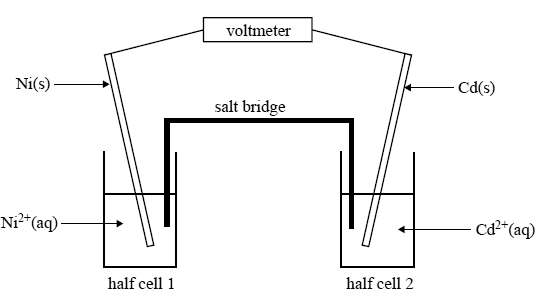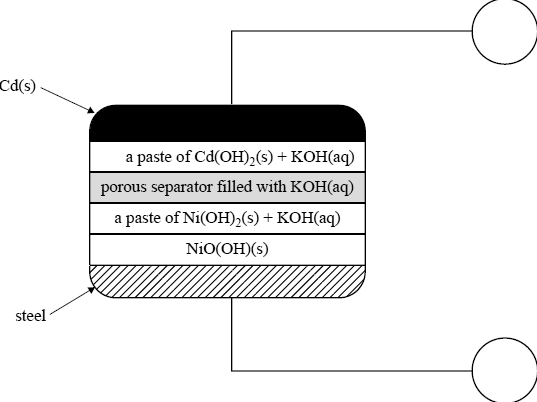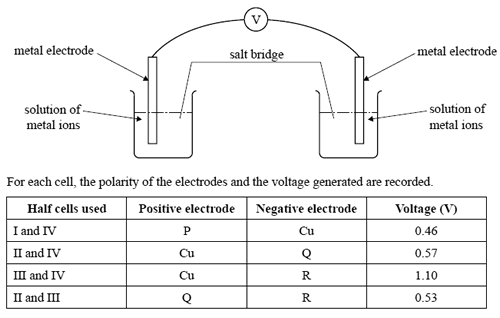Galvanic cells 2008 VCE A galvanic cell is constructed from the following two half cells under standard conditions. Half cell 1: a nickel electrode in a solution of 1.0 M nickel nitrate Half cell 2: a cadmium electrode in a solution of 1.0 M cadmium nitrate A sketch of the cell is given below.Given that the standard reduction potential of Cd2+(aq)/ Cd(s) is –0.40V, show on the above sketch the direction in which electrons will flow in the external circuit of this galvanic cell. Solution Give the equation for the half reaction that takes place at the anode of this cell. Solution List two factors that need to be considered when selecting an appropriate substance for use in the salt bridge. SolutionA rechargeable galvanic cell, also based on nickel and cadmium (NiCd cell), has been commercially available for a number of years and has been used to power small appliances such as mobile phones. A simplified diagram of a NiCd cell is given below.The overall cell reaction for the cell when discharging is Cd(s) + 2NiO(OH)(s) + 2H2O(l) → Cd(OH)2(s) + 2Ni(OH)2(s) Identify the positive and the negative electrodes by writing ‘+’ or ‘–’ in the circles provided in the diagram. Solution What feature of this secondary cell enables it to be recharged? Solution Give the equation for the half reaction that takes place at the negative electrode when the cell is discharging. Solution Give the equation for the half reaction that takes place at the electrode connected to the negative terminal of the power supply when the cell is recharging. SolutionFour half cells are constructed as follows. Half cell I: an electrode of metal P in a 1.0 M solution of P+(aq) ions Half cell II: an electrode of metal Q in a 1.0 M solution of Q+(aq) ions Half cell III: an electrode of metal R in a 1.0 M solution of R+(aq) ions Half cell IV: an electrode of Cu(s) metal in a 1.0 M solution of Cu2+(aq) ions The half cells are connected in pairs, as shown below, to form a series of galvanic cells.Which one of the following alternatives lists the metals in order of increasing strength as reductants? A. R, Q, Cu, P B. Cu, P, Q, R C. P, Cu, R, Q D. P, Cu, Q, R Solution